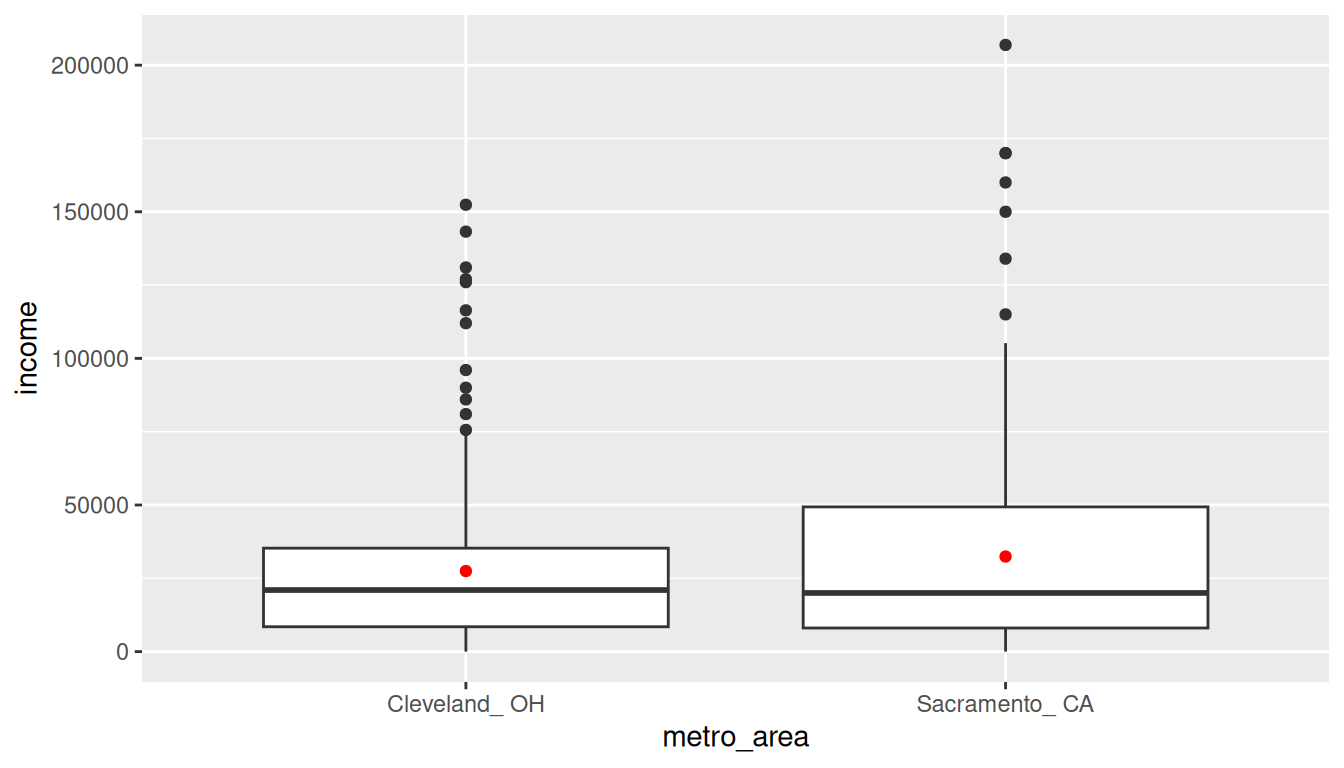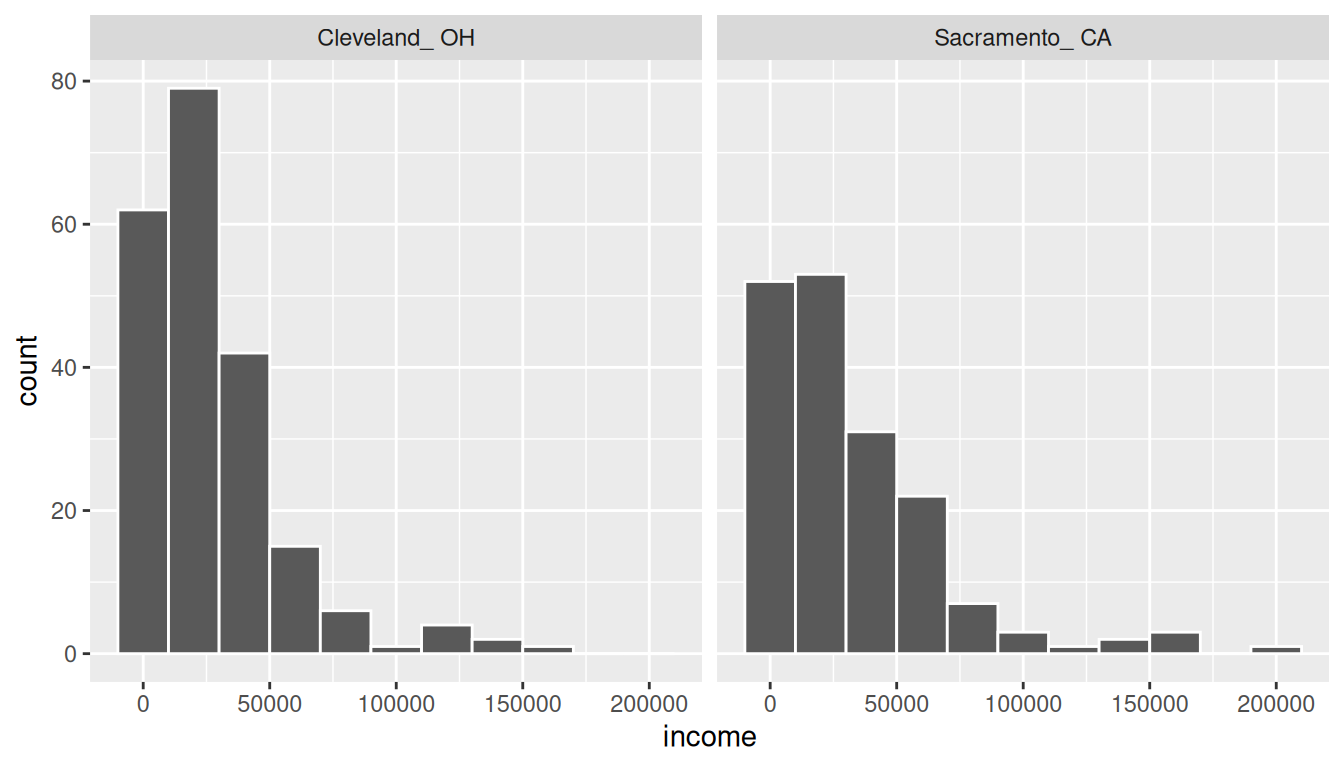## B.5 Two means (independent samples)

### B.5.1 Problem statement

Average income varies from one region of the country to another, and it often reflects both lifestyles and regional living expenses. Suppose a new graduate is considering a job in two locations, Cleveland, OH and Sacramento, CA, and he wants to see whether the average income in one of these cities is higher than the other. He would like to conduct a hypothesis test based on two randomly selected samples from the 2000 Census. (Tweaked a bit from Diez, Barr, and Çetinkaya-Rundel 2014 [Chapter 5])

### B.5.2 Competing hypotheses

#### In words

• Null hypothesis: There is no association between income and location (Cleveland, OH and Sacramento, CA).
• Alternative hypothesis: There is an association between income and location (Cleveland, OH and Sacramento, CA).

#### Another way in words

• Null hypothesis: The mean income is the same for both cities.
• Alternative hypothesis: The mean income is different for the two cities.

#### In symbols (with annotations)

• $$H_0: \mu_{sac} = \mu_{cle}$$ or $$H_0: \mu_{sac} - \mu_{cle} = 0$$, where $$\mu$$ represents the average income.
• $$H_A: \mu_{sac} - \mu_{cle} \ne 0$$

#### Set $$\alpha$$

It’s important to set the significance level before starting the testing using the data. Let’s set the significance level at 5% here.

### B.5.3 Exploring the sample data

cle_sac <- read.delim("https://moderndive.com/data/cleSac.txt") %>%
rename(
metro_area = Metropolitan_area_Detailed,
income = Total_personal_income
) %>%
na.omit()
inc_summ <- cle_sac %>%
group_by(metro_area) %>%
summarize(
sample_size = n(),
mean = mean(income),
sd = sd(income),
minimum = min(income),
lower_quartile = quantile(income, 0.25),
median = median(income),
upper_quartile = quantile(income, 0.75),
max = max(income)
)
kable(inc_summ) %>%
kable_styling(
font_size = ifelse(is_latex_output(), 10, 16),
latex_options = c("hold_position")
)
metro_area sample_size mean sd minimum lower_quartile median upper_quartile max
Cleveland_ OH 212 27467 27681 0 8475 21000 35275 152400
Sacramento_ CA 175 32428 35774 0 8050 20000 49350 206900

The boxplot below also shows the mean for each group highlighted by the red dots.

ggplot(cle_sac, aes(x = metro_area, y = income)) +
geom_boxplot() +
stat_summary(fun.y = "mean", geom = "point", color = "red")We are looking to see if a difference exists in the mean income of the two levels of the explanatory variable. Based solely on the boxplot, we have reason to believe that no difference exists. The distributions of income seem similar and the means fall in roughly the same place.

#### Collecting summary info

We now compute the observed statistic:

d_hat <- cle_sac %>%
specify(income ~ metro_area) %>%
calculate(
stat = "diff in means",
order = c("Sacramento_ CA", "Cleveland_ OH")
)
d_hat
# A tibble: 1 x 1
stat
<dbl>
1 4960.48

#### Randomization for hypothesis test

In order to look to see if the observed sample mean for Sacramento of 27467.066 is statistically different than that for Cleveland of 32427.543, we need to account for the sample sizes. Note that this is the same as looking to see if $$\bar{x}_{sac} - \bar{x}_{cle}$$ is statistically different than 0. We also need to determine a process that replicates how the original group sizes of 212 and 175 were selected.

We can use the idea of randomization testing (also known as permutation testing) to simulate the population from which the sample came (with two groups of different sizes) and then generate samples using shuffling from that simulated population to account for sampling variability.

set.seed(2018)
null_distn_two_means <- cle_sac %>%
specify(income ~ metro_area) %>%
hypothesize(null = "independence") %>%
generate(reps = 10000) %>%
calculate(
stat = "diff in means",
order = c("Sacramento_ CA", "Cleveland_ OH")
)
null_distn_two_means %>% visualize()We can next use this distribution to observe our $$p$$-value. Recall this is a two-tailed test so we will be looking for values that are greater than or equal to 4960.477 or less than or equal to -4960.477 for our $$p$$-value.

null_distn_two_means %>%
visualize(obs_stat = d_hat, direction = "both")##### Calculate $$p$$-value
pvalue <- null_distn_two_means %>%
get_pvalue(obs_stat = d_hat, direction = "both")
pvalue
# A tibble: 1 x 1
p_value
<dbl>
1  0.1262

So our $$p$$-value is 0.126 and we fail to reject the null hypothesis at the 5% level. You can also see this from the histogram above that we are not very far into the tail of the null distribution.

#### Bootstrapping for confidence interval

We can also create a confidence interval for the unknown population parameter $$\mu_{sac} - \mu_{cle}$$ using our sample data with bootstrapping. Here we will bootstrap each of the groups with replacement instead of shuffling. This is done using the groups argument in the resample function to fix the size of each group to be the same as the original group sizes of 175 for Sacramento and 212 for Cleveland.

boot_distn_two_means <- cle_sac %>%
specify(income ~ metro_area) %>%
generate(reps = 10000) %>%
calculate(
stat = "diff in means",
order = c("Sacramento_ CA", "Cleveland_ OH")
)
ci <- boot_distn_two_means %>%
get_ci()
ci
# A tibble: 1 x 2
2.5% 97.5%
<dbl>   <dbl>
1 -1359.50 11499.7
boot_distn_two_means %>%
visualize(endpoints = ci, direction = "between")We see that 0 is contained in this confidence interval as a plausible value of $$\mu_{sac} - \mu_{cle}$$ (the unknown population parameter). This matches with our hypothesis test results of failing to reject the null hypothesis. Since zero is a plausible value of the population parameter, we do not have evidence that Sacramento incomes are different than Cleveland incomes.

Interpretation: We are 95% confident the true mean yearly income for those living in Sacramento is between 1359.5 dollars smaller to 11499.69 dollars higher than for Cleveland.

Note: You could also use the null distribution based on randomization with a shift to have its center at $$\bar{x}_{sac} - \bar{x}_{cle} = \4960.48$$ instead of at 0 and calculate its percentiles. The confidence interval produced via this method should be comparable to the one done using bootstrapping above.

##### Check conditions

Remember that in order to use the short-cut (formula-based, theoretical) approach, we need to check that some conditions are met.

1. Independent observations: The observations are independent in both groups.

This metro_area variable is met since the cases are randomly selected from each city.

2. Approximately normal: The distribution of the response for each group should be normal or the sample sizes should be at least 30.

ggplot(cle_sac, aes(x = income)) +
geom_histogram(color = "white", binwidth = 20000) +
facet_wrap(~metro_area)We have some reason to doubt the normality assumption here since both the histograms show deviation from a normal model fitting the data well for each group. The sample sizes for each group are greater than 100 though so the assumptions should still apply.

1. Independent samples: The samples should be collected without any natural pairing.

There is no mention of there being a relationship between those selected in Cleveland and in Sacramento.

### B.5.6 Test statistic

The test statistic is a random variable based on the sample data. Here, we are interested in seeing if our observed difference in sample means ($$\bar{x}_{sac, obs} - \bar{x}_{cle, obs}$$ = 4960.477) is statistically different than 0. Assuming that conditions are met and the null hypothesis is true, we can use the $$t$$ distribution to standardize the difference in sample means ($$\bar{X}_{sac} - \bar{X}_{cle}$$) using the approximate standard error of $$\bar{X}_{sac} - \bar{X}_{cle}$$ (invoking $$S_{sac}$$ and $$S_{cle}$$ as estimates of unknown $$\sigma_{sac}$$ and $$\sigma_{cle}$$).

$T =\dfrac{ (\bar{X}_1 - \bar{X}_2) - 0}{ \sqrt{\dfrac{S_1^2}{n_1} + \dfrac{S_2^2}{n_2}} } \sim t (df = min(n_1 - 1, n_2 - 1))$ where 1 = Sacramento and 2 = Cleveland with $$S_1^2$$ and $$S_2^2$$ the sample variance of the incomes of both cities, respectively, and $$n_1 = 175$$ for Sacramento and $$n_2 = 212$$ for Cleveland.

#### Observed test statistic

Note that we could also do (ALMOST) this test directly using the t.test function. The x and y arguments are expected to both be numeric vectors here so we’ll need to appropriately filter our datasets.

cle_sac %>%
specify(income ~ metro_area) %>%
calculate(
stat = "t",
order = c("Cleveland_ OH", "Sacramento_ CA")
)
# A tibble: 1 x 1
stat
<dbl>
1 -1.50062

We see here that the observed test statistic value is around -1.5.

While one could compute this observed test statistic by “hand”, the focus here is on the set-up of the problem and in understanding which formula for the test statistic applies.

### B.5.7 Compute $$p$$-value

The $$p$$-value—the probability of observing an $$t_{174}$$ value of -1.501 or more extreme (in both directions) in our null distribution—is 0.13. This can also be calculated in R directly:

2 * pt(-1.501, df = min(212 - 1, 175 - 1), lower.tail = TRUE)
 0.135

We can also approximate by using the standard normal curve:

2 * pnorm(-1.501)
 0.133

Note that the 95 percent confidence interval given above matches well with the one calculated using bootstrapping.

### B.5.8 State conclusion

We, therefore, do not have sufficient evidence to reject the null hypothesis. Our initial guess that a statistically significant difference not existing in the means was backed by this statistical analysis. We do not have evidence to suggest that the true mean income differs between Cleveland, OH and Sacramento, CA based on this data.

### B.5.9 Comparing results

Observing the bootstrap distribution and the null distribution that were created, it makes quite a bit of sense that the results are so similar for traditional and non-traditional methods in terms of the $$p$$-value and the confidence interval since these distributions look very similar to normal distributions. The conditions also being met leads us to better guess that using any of the methods whether they are traditional (formula-based) or non-traditional (computational-based) will lead to similar results.

### References

Diez, David M, Christopher D Barr, and Mine Çetinkaya-Rundel. 2014. Introductory Statistics with Randomization and Simulation. First. Scotts Valley, CA: CreateSpace Independent Publishing Platform. https://www.openintro.org/stat/textbook.php?stat_book=isrs.